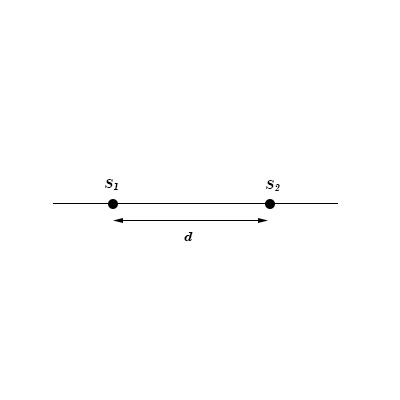# The top view diagram at right illustrates two point sources, S_1, and S_2. 1. On the diagram,...

## Question:

The top view diagram at right illustrates two point sources, {eq}S_1 {/eq}, and {eq}S_2 {/eq}.

1. On the diagram, indicate points for which the value of {eq}\Delta D {/eq} is:

i) largest and

ii) smallest. ({eq}\Delta D {/eq} is the difference in distances to the sources.)

2. What are the largest and smallest values of {eq}\Delta D {/eq} for this situation? Explain your reasoning.## Distance:

Distance is a physical variable. The measurement that used to find that how a point is far from a fixed point is known as distance. The distance between two points is calculated or measured in meters.

(a)

Given Data

• The difference in distances to the sources is {eq}\Delta D {/eq}.
• The distance between two points sources {eq}S_1 {/eq} and {eq}S_2 {/eq} is {eq}d {/eq}.

Let a point {eq}Q {/eq} is a point on the line extended {eq}{S_1}{S_2} {/eq} for which the distance between the {eq}{S_1}Q {/eq} and {eq}{S_2}Q {/eq} is maximum.

So,

{eq}\begin{align*} \Delta {D_{\max }} &= {S_1}Q - {S_2}Q\\ &= d \end{align*} {/eq}

Thus, point {eq}Q {/eq} is the point for which the values of {eq}\Delta D {/eq} is largest.

Let {eq}PM {/eq} is the perpendicular bisector of the line {eq}{S_1}{S_2} {/eq}.

{eq}{S_1}P {/eq} and {eq}{S_2}P {/eq} is the distance of point {eq}P {/eq} from the source. As point {eq}P {/eq} is on the perpendicular of distance {eq}d {/eq}, the distances {eq}{S_1}P {/eq} and {eq}{S_2}P {/eq} will be equal.

So,

{eq}\begin{align*} {{S_1}P} &= {{S_2}P}\\ {{S_1}P} - {{S_2}P} &= 0\\ \Delta{D_{min}} &= 0 \end{align*} {/eq}

Thus, point {eq}P {/eq} is the point for which the values of {eq}\Delta D {/eq} is smallest.

(b)

As the distance of sources from point on the perpendicular bisector of {eq}{S_1}{S_2} {/eq} is equal, the value of {eq}\Delta D {/eq} is 0. For any point which is on line extended from line {eq}{S_1}{S_2} {/eq}, the value of {eq}\Delta D {/eq} is d which is maximum. Thus, from above calculation and discussion the largest and smallest values of {eq}\Delta D {/eq} is d and 0 respectively.